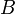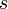Errata: Explanation of the lack of zeros when B or C is full rank is confusing

In the paragraph following equation (8.17), the text states that there are no zeros if either theormatrix is "full rank". This wording is misleading since a non-square matrix can be full rank without having fully independent rows (for) or columns (for). The text should read
Notice in particular that if the matrixhas full row rank, then the matrix in equation (8.17) haslinearly independent rows for all values of. Similarly there arelinearly independent columns if the matrixhas full column rank. This implies that systems where the matrixoris square and full rank do not have zeros. In particular it means that a system has no zeros if it is fully actuated\index{fully actuated systems} (each state can be controlled independently) or if the full state is measured.